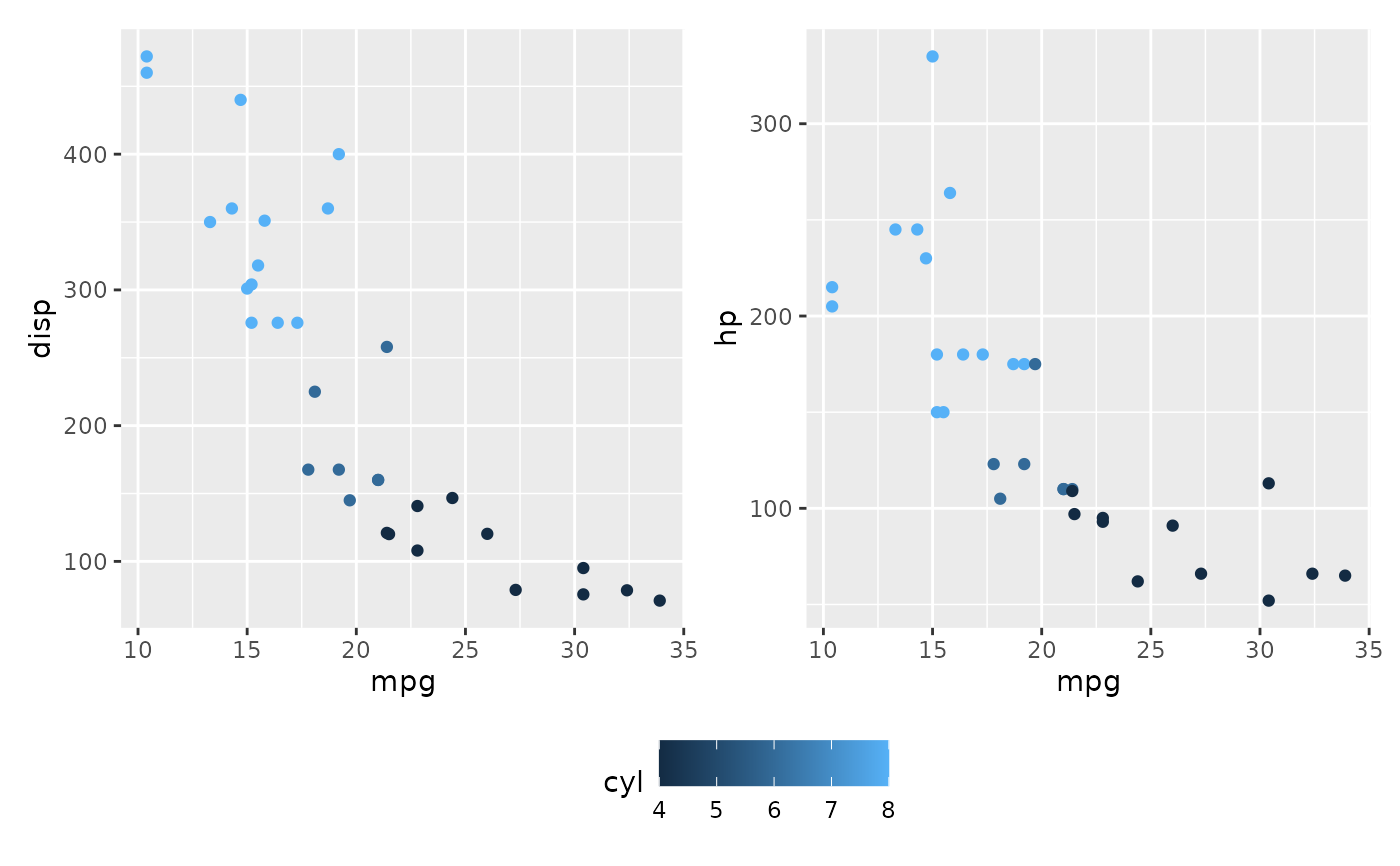In order to control how different plots are layed out, you need to add a layout specification. If you are nesting grids, the layout is scoped to the current nesting level.

plot_layout(
ncol = NULL,
nrow = NULL,
byrow = NULL,
widths = NULL,
heights = NULL,
guides = NULL,
tag_level = NULL,
design = NULL
)

## Arguments

ncol, nrow The dimensions of the grid to create - if both are NULL it will use the same logic as facet_wrap() to set the dimensions Analogous to byrow in matrix(). If FALSE the plots will be filled in in column-major order The relative widths and heights of each column and row in the grid. Will get repeated to match the dimensions of the grid. A string specifying how guides should be treated in the layout. 'collect' will collect guides below to the given nesting level, removing duplicates. 'keep' will stop collection at this level and let guides be placed alongside their plot. auto will allow guides to be collected if a upper level tries, but place them alongside the plot if not. If you modify default guide "position" with theme(legend.position=...) while also collecting guides you must apply that change to the overall patchwork (see example). A string ('keep' or 'new') to indicate how auto-tagging should behave. See plot_annotation(). Specification of the location of areas in the layout. Can either be specified as a text string or by concatenating calls to area() together. See the examples for further information on use.

## Value

A plot_layout object to be added to a ggassmble object

## Examples

library(ggplot2)

p1 <- ggplot(mtcars) + geom_point(aes(mpg, disp))
p2 <- ggplot(mtcars) + geom_boxplot(aes(gear, disp, group = gear))
p3 <- ggplot(mtcars) + geom_bar(aes(gear)) + facet_wrap(~cyl)
p4 <- ggplot(mtcars) + geom_bar(aes(carb))
p5 <- ggplot(mtcars) + geom_violin(aes(cyl, mpg, group = cyl))

# The plots are layed out automatically by default
p1 + p2 + p3 + p4 + p5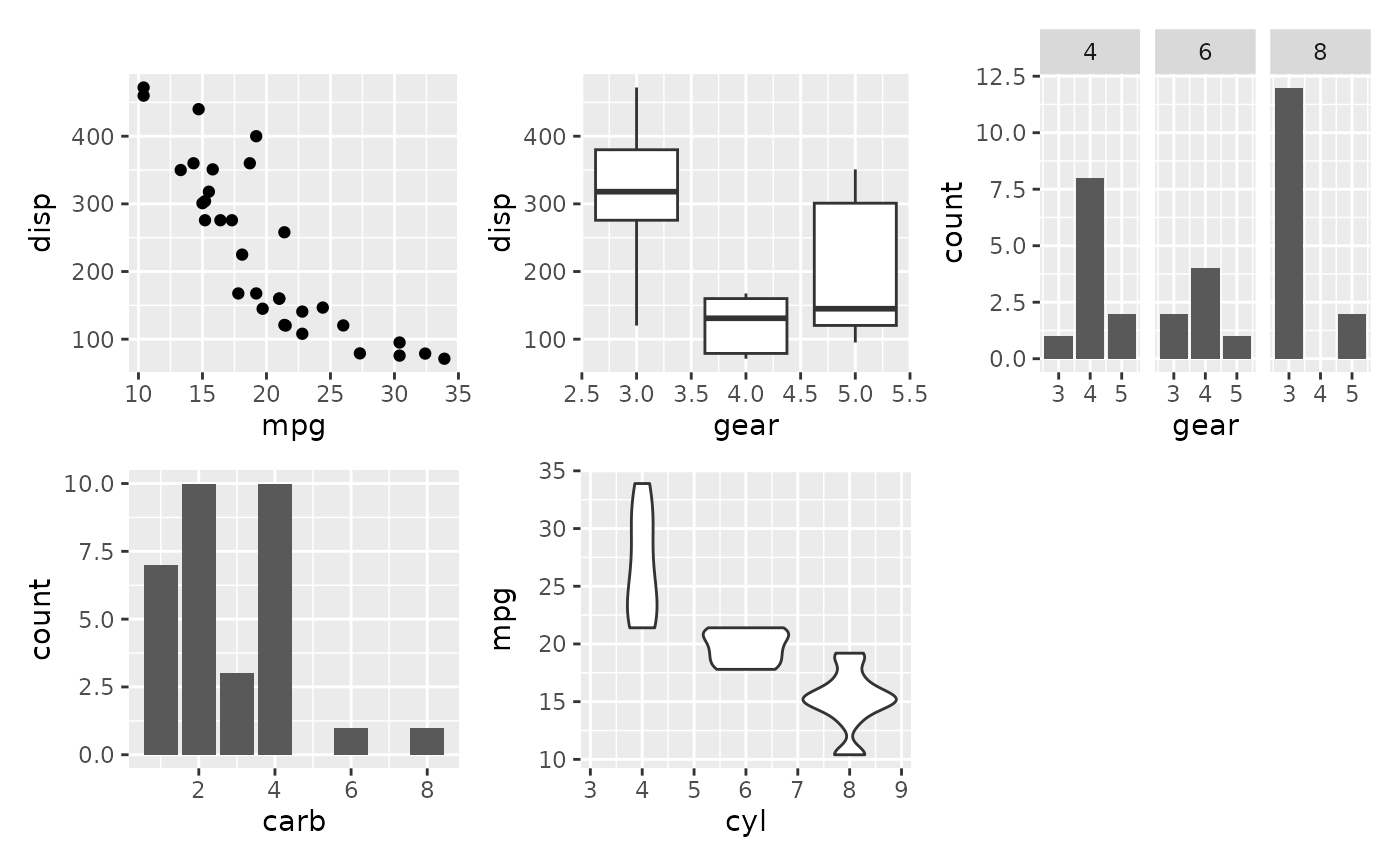# Use byrow to change how the grid is filled out
p1 + p2 + p3 + p4 + p5 + plot_layout(byrow = FALSE)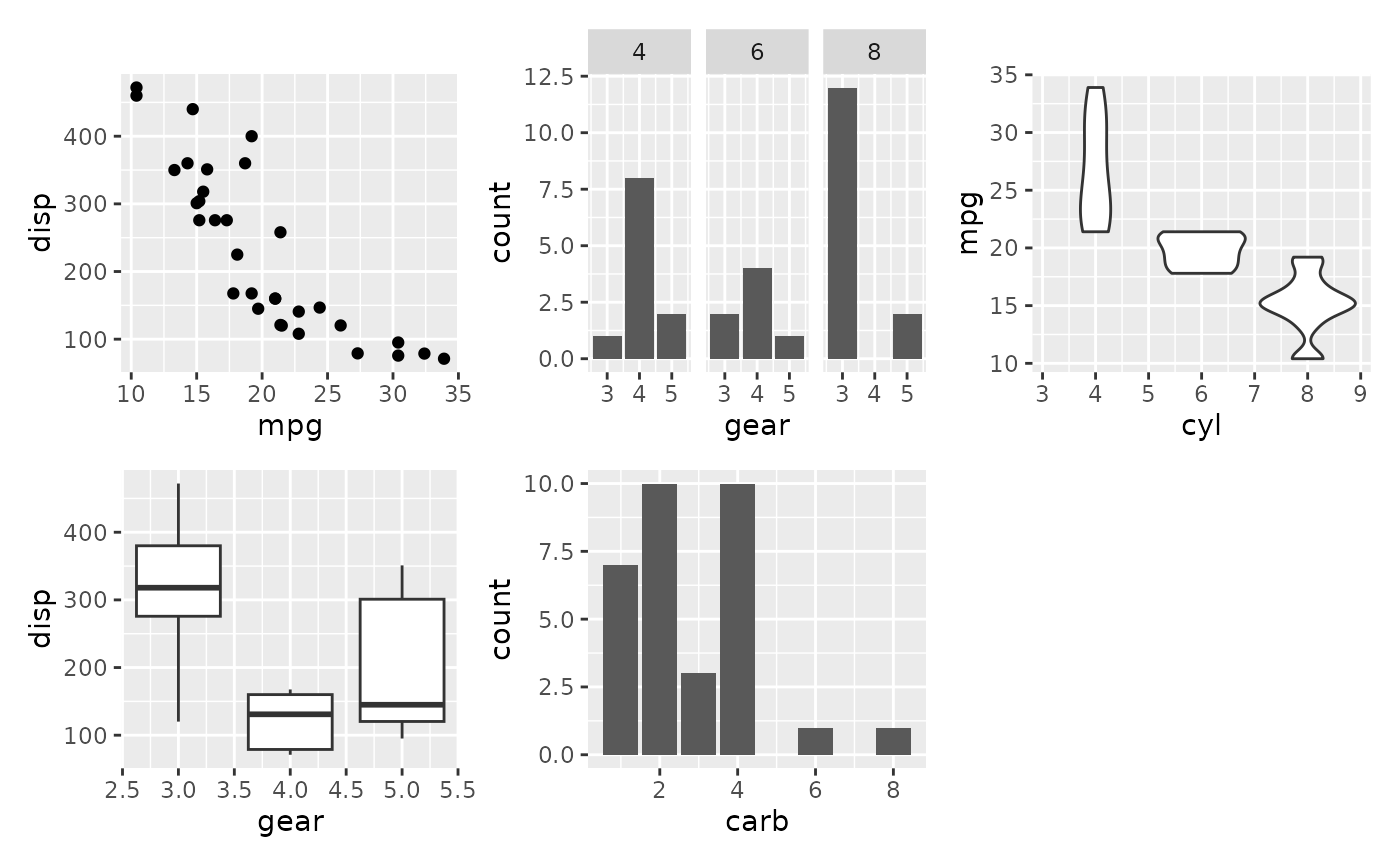# Change the grid dimensions
p1 + p2 + p3 + p4 + p5 + plot_layout(ncol = 2, widths = c(1, 2))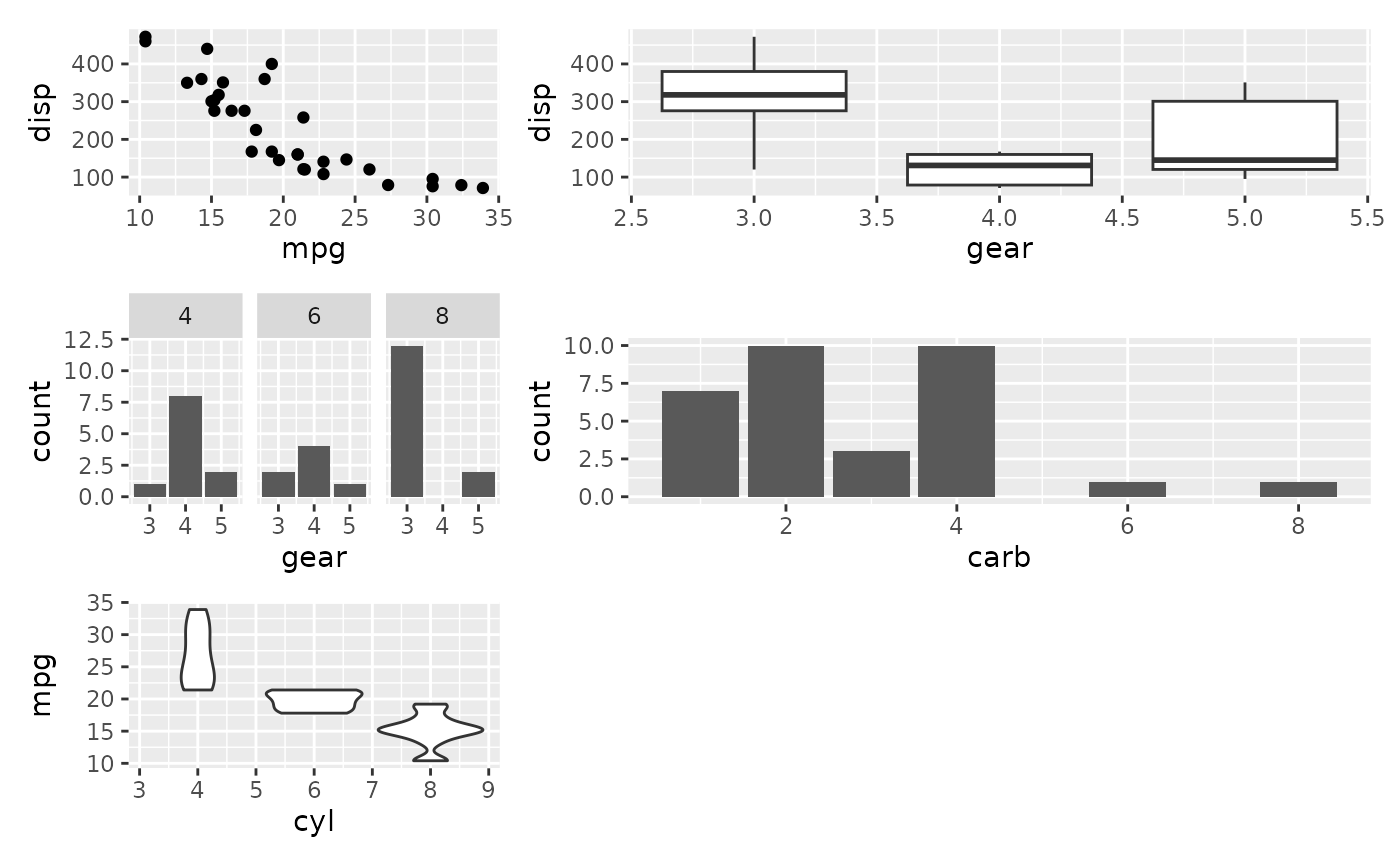# Define layout at different nesting levels
p1 +
p2 +
(p3 +
p4 +
plot_layout(ncol = 1)
) +
p5 +
plot_layout(widths = c(2, 1))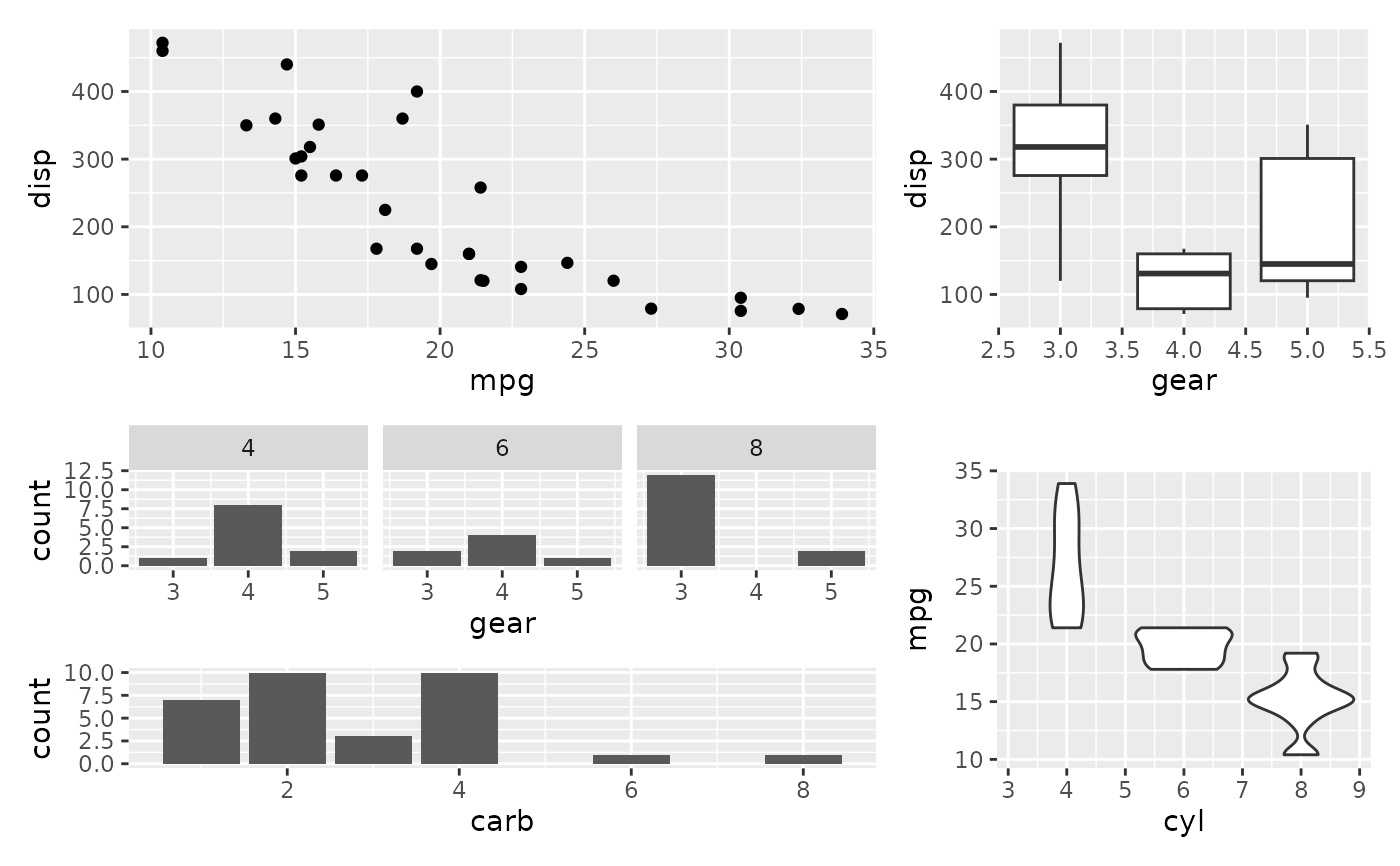# Complex layouts can be created with the design argument
design <- c(
area(1, 1, 2),
area(1, 2, 1, 3),
area(2, 3, 3),
area(3, 1, 3, 2),
area(2, 2)
)
p1 + p2 + p3 + p4 + p5 + plot_layout(design = design)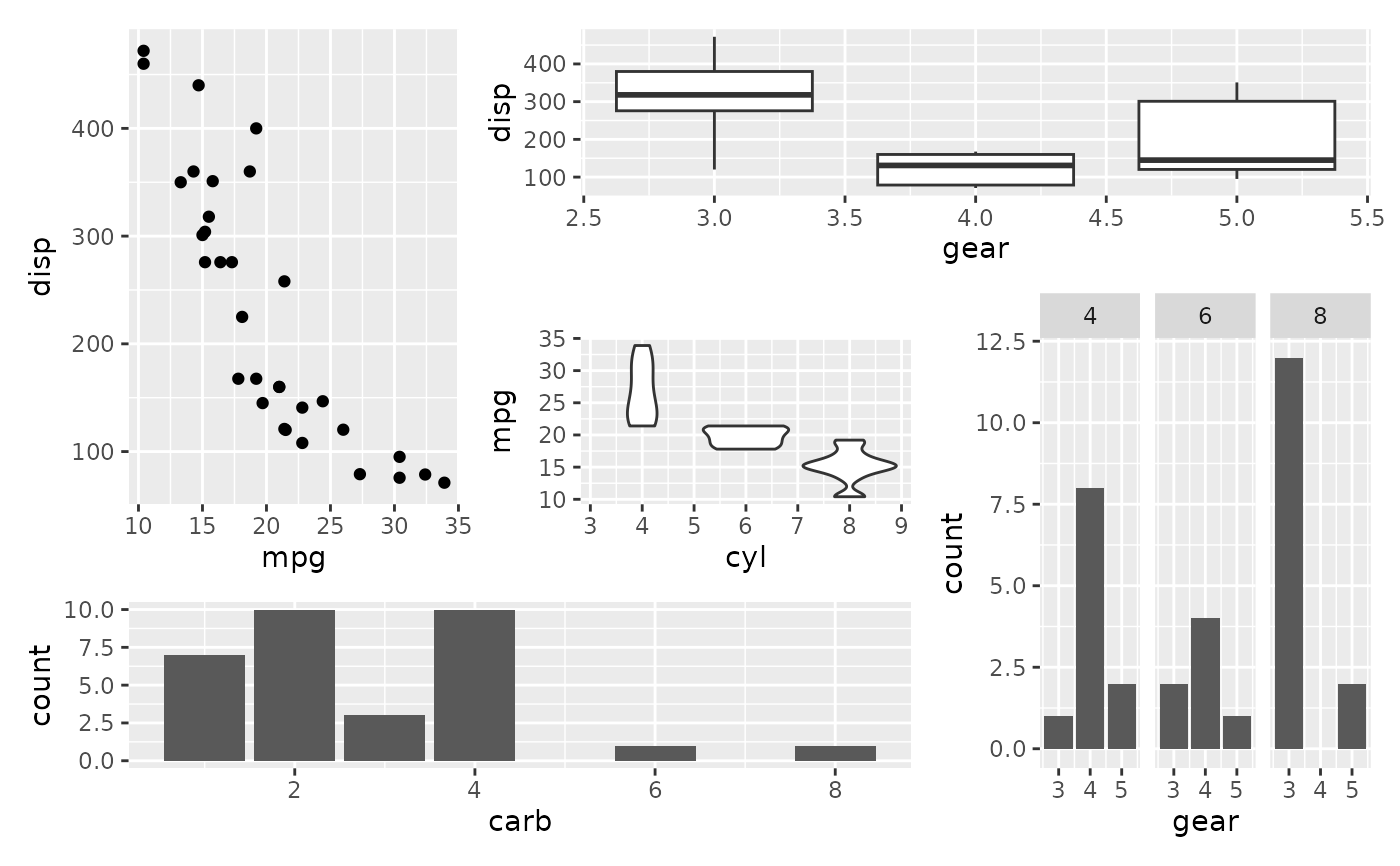# \donttest{
# The same can be specified as a character string:
design <- "
122
153
443
"
p1 + p2 + p3 + p4 + p5 + plot_layout(design = design)# When using strings to define the design # can be used to denote empty
# areas
design <- "
1##
123
##3
"
p1 + p2 + p3 + plot_layout(design = design)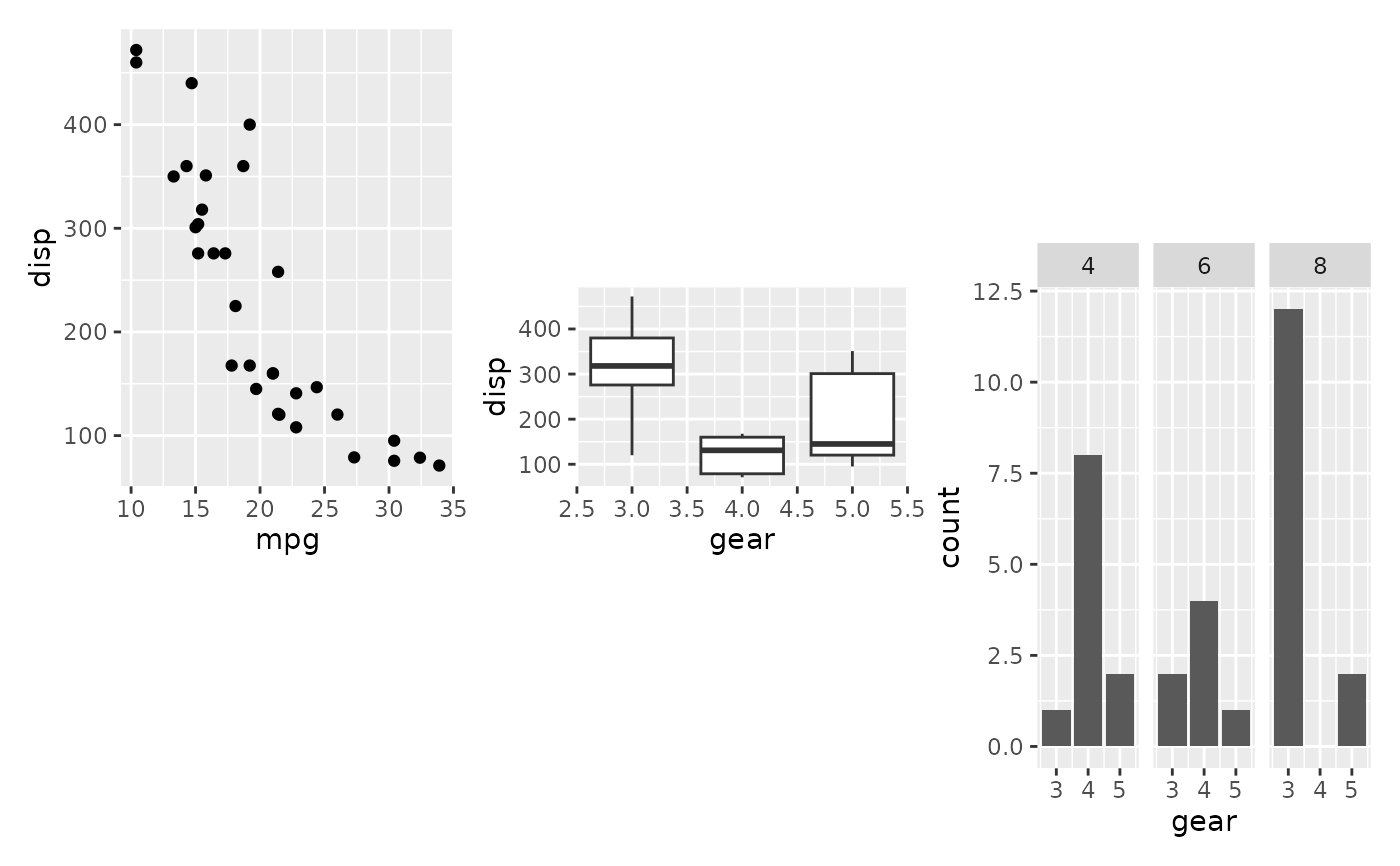# }
# Use guides="collect" to remove duplicate guides
p6 <- ggplot(mtcars) + geom_point(aes(mpg, disp, color=cyl))
p7 <- ggplot(mtcars) + geom_point(aes(mpg, hp, color=cyl))
p6 + p7 + plot_layout(guides='collect')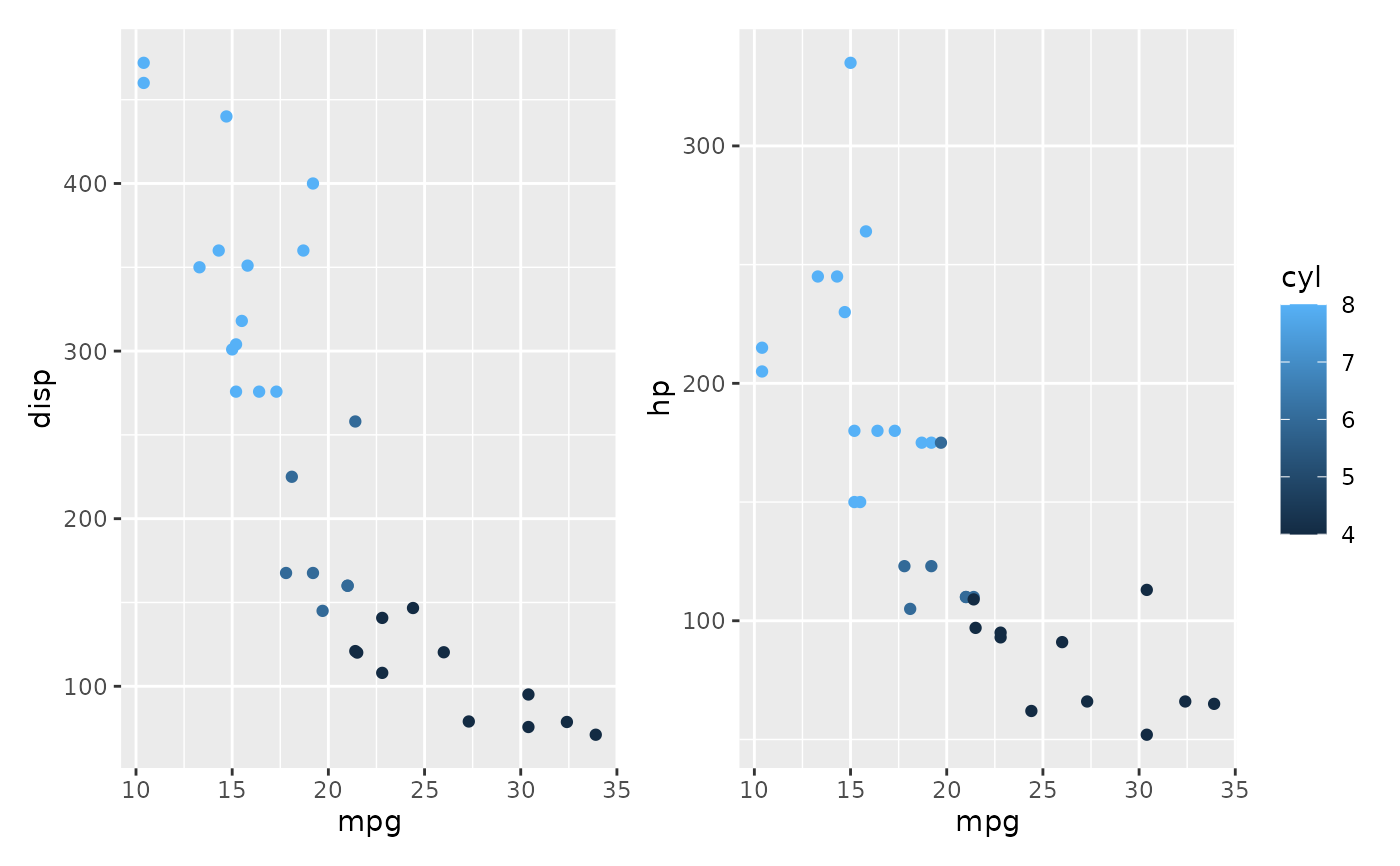# Guide position must be applied to entire patchwork
p6 + p7 + plot_layout(guides='collect') &
theme(legend.position='bottom')